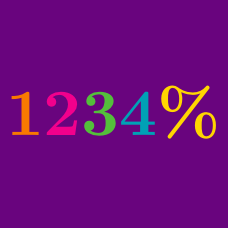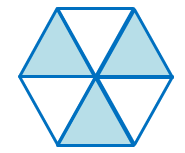Everyday Math

# Converting Decimals and Percentages

Convert the decimal $0.61$ to a percentage.

Details and assumptions

If your answer is $12\%$, type it in as $12$.

The fraction of the polygon painted below is $X.$ What is $25\%$ of $X?$Which of the following is equal to the product of the proportion of stars and the proportion of pentagons to all polygons below?Details and assumptions:

• Each polygon is counted as one regardless of how big or what kind it is.

Which of the following is equal to $0.1 \times 0.5 + 0.37$?

Convert $8\% \div 32\%$ to a decimal.

×